Next: 6.5.3 Comparison with the Up: 6.5 Discussion Previous: 6.5.1 Performance

## 6.5.2 Limitations

There are two sources of limitations of the differential method, namely, computational requirements that are getting too large and failure of the stabilized march technique due to strongly damped solution modes resulting in numerical instabilities. The first can be overcome by an increase of the available computer resources, e.g., with the next computer generation it will be possible to solve problems of double size. The second, however, is a principle limitation and thus more serious. We will discuss both impacts from a qualitative point of view.

Computational Requirements. In (6.77) is shown that the computational costs and storage demands grow with the second and the third orders of the number NODE of the Fourier coefficients considered, respectively. Since NODE is determined by the two truncation frequencies Nx and Ny of the Fourier expansion of the lateral field components (cf. (6.29)), they should be chosen as small as possible. The physical impacts of the truncation cause a low-pass filtering of the field as well as of the geometry and have already been discussed in Section 6.2.5. An analytical investigation of a lateral stepped permittivity including error-bounds is presented in Appendix D. Hence, there is a fundamental tradeoff between computational requirements and accuracy. To be more precise we have to answer the question of how many Fourier modes are required to represent the EM field with a precision that is high enough. For that we reconsider the sampling of the lateral wavevectors introduced in (6.13)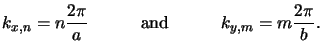(6.52)

The following considerations are performed for a homogeneous medium only. However, the results are useful for a qualitative study of a general inhomogeneous medium. Denoting the permittivity of the medium by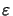=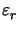+ jthe vertical wavevector component writes to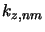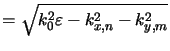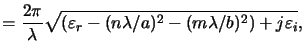(6.53)

whereby k0 = 2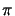/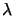is inserted for the wavenumber. Since the vertical dependence Enm(z) of the EM field is an exponential one, i.e., we have plane waves due to the homogeneity and thus Enm(z)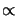exp(jkz, nmz), it is described by a damped oscillating function like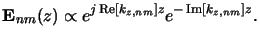(6.54)

The oscillation period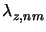and the penetration depth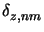are simply given by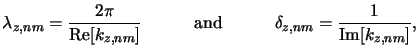(6.55)

whereby the real and imaginary parts of kz, nm are calculated from (6.79) to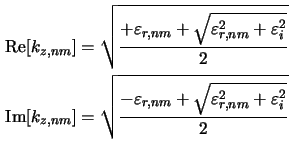with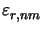defined as the real part of (cf. (6.79))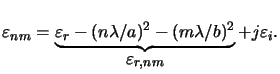(6.56)

We are now interested in the minimal values of both, the oscillation periodand the penetration depth, depending on the index pair (n, m). This means that we have to determine (n, m) for which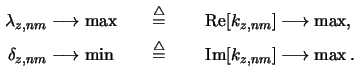Note that minimal penetration depth means maximal damping. From (6.82) we see that Re[kz, nm] is a monotone increasing function whereas Im[kz, nm] is a monotone decreasing function with respect to, i.e.,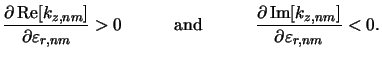(6.57)

The extreme values are thus given by (cf. (6.84))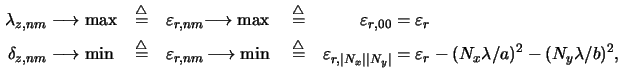whereby Nx and Ny are the truncation frequencies of the Fourier expansions of the EM field. The mode (0, 0) propagating in vertical direction exhibits the highest oscillation and the least damping, whereas the four most oblique ones (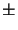Nx,Ny) are least oscillating but strongest damped--a result that is physically reasonable. Hence the oscillating nature of the EM field is reflected by the ODE system independently of the truncation frequencies. The truncation frequencies have to be chosen in a way that also damped modes are resembled with sufficient precision. But the higher Nx and/or Ny are, the stronger the damping becomes, which causes stability problems of the algorithm. Before we consider this problem another consequence from (6.86) shall be discussed. As can be seen the ratio between wavelengthand the lateral dimensions a and b of the simulation domain determines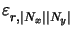and thus also the imaginary part Im[kz,| Nx|| Ny|] of the vertical wavevector component (cf. (6.82)). These ratios are the critical quantities for the choice of appropriate truncation frequencies, i.e.,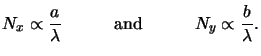(6.58)

The rank of the resulting ODE system and thus the computational requirements are determined by it. For example, in the simulation results presented in Chapter 8 this ratio was approximately four, i.e., for 248 nm lithography the lateral extensions were 1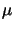m. This shows that the differential method is only suited for single feature simulation, a situation that applies to all rigorous EM field solvers.

Numerical Instabilities. Numerical instabilities are caused by damped solution modes. The preceding discussion shows that there are two reasons for the existence of such modes, namely, a strongly absorptive medium and high truncation frequencies. The first is self-evident, whereas the second results from the fact that for larger Nx and/or Ny the real partof the permittivitybecomes negative (cf. (6.83)). These modes are then called evanescent since in a loss-less medium with= 0 they propagate in lateral direction and are damped vertically, i.e., in the orthogonal direction to the propagation direction. In a lossy or absorbing medium with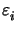> 0 the real part Re[kz, nm] of the vertical component of the wavevector almost vanishes whereas the negative part Im[kz, nm] becomes dominating. In the limiting case | Nx|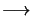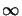and | Ny|we have Re[kz, nm]0 and/or Im[kz, nm]. This situation is graphically illustrated in Figure 6.6. For a proper function of the stabilized march algorithm the truncation frequencies have to be chosen carefully so that all propagating modes are accounted for, but at most only a few evanescent ones are considered.

The discussion shows that the truncation of the EM field expansion is crucial for the operation of the differential method--not just for the computational requirements but also for the convergence of the algorithm. The same situation applies to the waveguide model, since it it is actually a special case of the differential method as will be shown in the next section.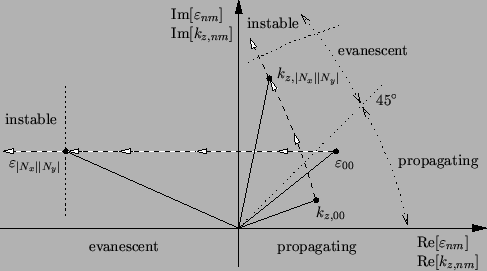Next: 6.5.3 Comparison with the Up: 6.5 Discussion Previous: 6.5.1 Performance
Heinrich Kirchauer, Institute for Microelectronics, TU Vienna
1998-04-17